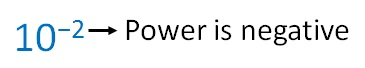Suppose we have number likeHere,

Exponent(Power) is negative

It means

10 − 2 = 1/10 2

= 1/100

= 001/100

= 0.01

∴ 10 −2 = 0.01

10 − 4 = 1/10 4

= 1/10000

= 00001/10000

= 0.0001

2 − 4 = 1/2 4

= 1/(2 × 2 × 2 × 2)

= 1/(4 × 4)

= 1/16

3 − 2 = 1/3 2

= 1/(3 × 3)

= 1/9

6 − 3 = 1/6 3

= 1/(6 × 6 × 6)

= 1/(36 × 6)

= 1/216

(1/3) -2 = 1 -2 /3 -2

= 1/3 -2

= 3 2

= 9

(1/ 10) - 4 = 1 -4 /10 -4

= 1/10 -4

= 10 4

= 10000

( 2 / 3) - 3 = 2 -3 /3 -3

= ((1/2 3 ))/((1/3 3 ) )

= 1/2 3  ÷ 1/3 3

= 1/2 3  × 3 3 /1

= 3 3 /2 3

= (3 × 3 × 3)/(2 × 2 × 2)

= (9 × 3)/(4 × 2)

= 27/8

1. Chapter 12 Class 8 Exponents and Powers
2. Concept wise
3. Exponents

Exponents